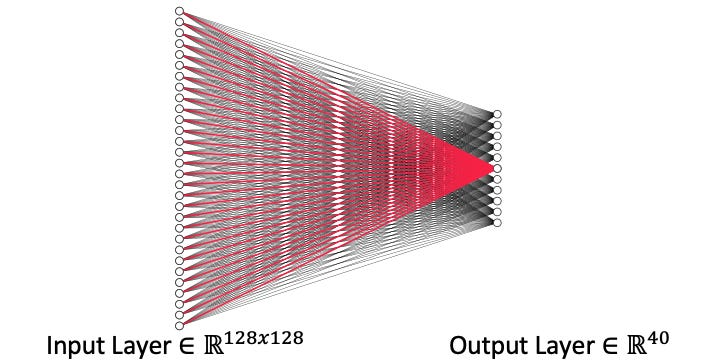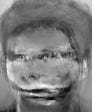# Uncovering a model’s secrets (Model Inversion Part I)

Source: Deep Learning on Medium

Have you ever woken up in the middle of the night after having a terrifying dream that your boss found that embarrassing Facebook picture of you in high school with a blue mohawk? You immediately go to facebook and delete the photo and think all is well. Well I’m here to tell you that it may not be the case. Facebook, and other image sharing sites, use machine learning algorithms to process user images and these algorithms have tendency to memorize certain elements of their training data, including that blue mohawk. As long as the model is accessible, the data -in this case your embarrassing image — could be as well.

Lab41 recently started tackling the question of how much information is a model memorizing and how can we better understand this? Let’s begin by looking at a very simple example classifying two clusters in 2D with x and y value pairs and how a model memorizes the average of the dataset. These two groups come from Gaussian distributions with means (-2,0) and (2,0).

We classify these points using a very simple fully connected model with two inputs (x and y coordinate) and two classification outputs. This model is simply a 2 by 2 matrix, with one weight corresponding to each class-feature permutation.

During training, the weights of this simple model are updated through gradient descent. Each time we update our weights, they are being updated by the gradient which is equal to the dataset for this model. This reliance on the training data to shape model weights should sound concerning. It’s the fundamental source of memorization in ML models. Maybe you are thinking that it is just the updated gradients and not the model that are based on the dataset, well this is only partially true. The model is not becoming the dataset, but it is being modified directly by the dataset.

What does this mean for our simple toy example? It means that we are updating our weights at the rate of the average of each class. w1,1 is being updated at the rate of the average x value of the orange class, w1,2 is being updated at the rate of the average x value of the blue class and so on for the rest of the weights. Below we can see how the weights change through our training process. w1,1 continues increasing at the same rate that w1,2 decreases, as a result of the ratio of the average of these two classes being 1:-1.

So our weights end up memorizing the average ratio between features and classes, but the magnitudes are unconstrained. After 10,000 iterations the weights are w1,1 = 5.27 w1,2 = -5.25 w1,2 = 0.01 and w2,2 = 0.01 not really reflecting the average which would be something like w1,1 = 2 w1,2 = -2 w1,2 = 0 and w2,2 = 0. But all this requires is a normalization, something as simple as knowing that the maximum of the dataset is 2. We normalize our weights to the max of the dataset and our weights are now the average of the dataset, w1,1 = 2 w1,2 = -2 w1,2 = 0 and w2,2 = 0. Ok so our toy example memorized some toy Gaussian averages…big deal. Well let’s see what happens with image data.

Image memorization

For image data we will start by using the ATT dataset. The ATT dataset is a very small dataset of 400 images with 40 individuals, which is 10 images per person. The classification task here is to classify the images of the 40 individuals respectively. The classification model is again a small fully connected model that takes each pixel and maps it to a class as shown below.Image classification model, red weights correspond to the class of interest

As we train our models, the weights are once again being modified directly by the input data. The red weights in the figure are the weights that correspond to a particular class. These weights are changed by the images corresponding to that one class. What does this look like? well if we reshape the red weights in our model back into an image, they look like the dataset. Below are three examples of three different class weights reshaped into an image.Weights of model for three different classes reshaped to an image

No need for a fancy model inversion attack, simply reshaping the model’s weights and normalizing to 255 and we get the average image of the class. I do want to emphasis that the model memorized the average of the class this is why the image above is blurry, and also why it doesn’t work as well with variations in pose like the classes in the middle and right.

The question now is how do we defend against these issues and fundamentally it comes down to what does the model need to know about the data to be accurate. By simply changing the optimization from SGD to ADAM the model weights stay close to the initialization values and still achieve 100% validation accuracy. To do this task the model doesn’t need to know much about the dataset and can still achieve high accuracy. By the weights staying close to the random initialization, the weights do not represent the dataset as well. This may lead to more generalization concerns but greatly decrease the privacy implications.